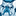# QlikView App Dev

Discussion Board for collaboration related to QlikView App Development.

Announcements
cancel
Showing results for
Did you mean:Not applicable

## Looping through Table Rows

I want to Loop through each row in a table, set variables to the values of each row in order to do some repetitive processing.  Seems like a simple construct that would be used often.  Here is what I thought would work, but for some reason the data coming back doesn't seem to match across the column for each row returned, and as I work through index by third iteration I'm getting nulls on the second column.

BOX:

"MRC", 49152
"TTN", 49152
"PLT", 12288
"GLD", 12288
"SVR", 12288
"IRN", 12288
]
;

LET NumRows=NoOfRows('BOX');
FOR i=1 to \$(NumRows)

LET vBox=FieldValue('BOXName',\$(i));

LET vVolCnt=FieldValue('VOLCnt',FieldIndex('BOXName','\$(vBox)'));
//do some stuff....
NEXT;

Result set for variables after run:

vBoxvVolCnt
MRC49152
TTN

12288

PLT<NULL>
GLD

<NULL>

SVR<NULL>
IRN<NULL>

Seems I'm missing something very elementary here13 RepliesNot applicable
Author

What about when you use the value from one to find the row in a different table?Employee

Was there any final conclusion on this one? I have an example where I did not use the third parameter, and I got unexpected results. To be safe, and like Henric says here and here, I will use a third parameter; the name of the Table where I expect to find that Field.Contributor

Great solution! Just the count of the variable i should start from 0:

BOX:

"MRC", 49152
"TTN", 49152
"PLT", 12288
"GLD", 12288
"SVR", 12288
"IRN", 12288
]
;

LET NumRows=NoOfRows('BOX')-1;
FOR I=0 to \$(NumRows)

LET vBox=Peek('BOXName',\$(i));

LET vVolCnt=Peek('VOLCnt',\$(i));//do some stuff....
NEXT;

Let vBox= Null();
Let NumRows= Null();
Let I = Null();Partner

Like if you still come to this thread to copy and paste the For loop instead of typing it out. 😁